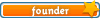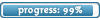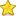Welcome

It is highly recommended that you have Javascript enabled; many features will not work unless you do. The recommended browsers are Firefox and Chrome; the board is also NOT mobile-friendly.

If you were referred by someone (ex. me, Jessica) please put their username in the referral box on the registration page. Ask them if you don't know their username.

If you are visiting for TESTING PURPOSES ONLY, this is the test account information:

Test

Select a forum to post in:

## Redox Reactions

Science topics

Moderators: Jessica, Teachers

Looking for a topic?
View the Science Topics Directory to find topics quicker under different kinds of science.

### Redox Reactions

Redox reactions, or oxidation-reduction reactions, have a number of similarities to acid-base reactions. Fundamentally, redox reactions are a family of reactions that are concerned with the transfer of electrons between species. Like acid-base reactions, redox reactions are a matched set -- you don't have an oxidation reaction without a reduction reaction happening at the same time. Oxidation refers to the loss of electrons, while reduction refers to the gain of electrons. Each reaction by itself is called a "half-reaction", simply because we need two (2) half-reactions to form a whole reaction. In notating redox reactions, chemists typically write out the electrons explicitly:

Cu (s) ----> Cu[super]2+[/super] + 2 e[super]-[/super]

This half-reaction says that we have solid copper (with no charge) being oxidized (losing electrons) to form a copper ion with a plus 2 charge. Notice that, like the stoichiometry notation, we have a "balance" between both sides of the reaction. We have one (1) copper atom on both sides, and the charges balance as well. The symbol "e-" represents a free electron with a negative charge that can now go out and reduce some other species, such as in the half-reaction:

2 Ag[super]+[/super] (aq) + 2 e[super]-[/super] ------> 2 Ag (s)

Here, two silver ions (silver with a positive charge) are being reduced through the addition of two (2) electrons to form solid silver. The abbreviations "aq" and "s" mean aqueous and solid, respectively. We can now combine the two (2) half-reactions to form a redox equation:We can also discuss the individual components of these reactions as follows. If a chemical causes another substance to be oxidized, we call it the oxidizing agent. In the equation above, Ag+ is the oxidizing agent, because it causes Cu(s) to lose electrons. Oxidants get reduced in the process by a reducing agent. Cu(s) is, naturally, the reducing agent in this case, as it causes Ag+ to gain electrons.

As a summary, here are the steps to follow to balance a redox equation in acidic medium (add the starred step in a basic medium):

1. Divide the equation into an oxidation half-reaction and a reduction half-reaction
2. Balance these
• Balance the elements other than H and O
• Balance the O by adding H[sub]2[/sub]O
• Balance the H by adding H[super]+[/super]
• Balance the charge by adding e[super]-[/super]
3. Multiply each half-reaction by an integer such that the number of e- lost in one equals the number gained in the other
4. Combine the half-reactions and cancel
5. **Add OH[super]-[/super] to each side until all H[super]+[/super] is gone and then cancel again**

In considering redox reactions, you must have some sense of the oxidation number (ON) of the compound. The oxidation number is defined as the effective charge on an atom in a compound, calculated according to a prescribed set of rules. An increase in oxidation number corresponds to oxidation, and a decrease to reduction. The oxidation number of a compound has some analogy to the pH and pK measurements found in acids and bases -- the oxidation number suggests the strength or tendency of the compound to be oxidized or reduced, to serve as an oxidizing agent or reducing agent. The rules are shown below. Go through them in the order given until you have an oxidation number assigned.

1. For atoms in their elemental form, the oxidation number is 0
2. For ions, the oxidation number is equal to their charge
3. For single hydrogen, the number is usually +1 but in some cases it is -1
4. For oxygen, the number is usually -2
5. The sum of the oxidation number (ONs) of all the atoms in the molecule or ion is equal to its total charge.

As a side note, the term "oxidation", with its obvious root from the word "oxygen", assumes that oxygen has an oxidation number of -2. Using this as a benchmark, oxidation numbers were assigned to all other elements. For example, if we look at H[sub]2[/sub]O, and assign the value of -2 to the oxygen atom, the hydrogens must each have an oxidation number of +1 by default, since water is a neutral molecule. As an example, what is the oxidation number of sulfur in sulfur dioxide (SO[sub]2[/sub])? Given that each oxygen atom has a -2 charge, and knowing that the molecule is neutral, the oxidation number for sulfur must be +4. What about for a sulfate ion (SO[sub]4[/sub] with a total charge of -2)? Again, the charge of all the oxygen atoms is 4 x -2 = -8. Sulfur must then have an oxidation number of +6, since +6 + (-8) = -2, the total charge on the ion. Since the sulfur in sulfate has a higher oxidation number than in sulfur dioxide, it is said to be more highly oxidized.

Working with redox reactions is fundamentally a bookkeeping issue. You need to be able to account for all of the electrons as they transfer from one species to another. There are a number of rules and tricks for balancing redox reactions, but basically they all boil down to dealing with each of the two half-reactions individually. Consider for example the reaction of aluminum metal to form alumina (Al[sub]2[/sub]O[sub]3[/sub]). The unbalanced reaction is as follows:Looking at each half reaction separately:This reaction shows aluminum metal being oxidized to form an aluminum ion with a +3 charge. The half-reaction below shows oxygen being reduced to form two (2) oxygen ions, each with a charge of -2.If we combine those two (2) half-reactions, we must make the number of electrons equal on both sides. The number 12 is a common multiple of three (3) and four (4), so we multiply the aluminum reaction by four (4) and the oxygen reaction by three (3) to get 12 electrons on both sides. Now, simply combine the reactions. Notice that we have 12 electrons on both sides, which cancel out. The final step is to combine the aluminum and oxygen ions on the right side using a cross multiply technique:Taking care of the number of atoms, you should end up with:• 0Jessica
Board Owner
Topic AuthorSapphirePosts: 3,470
Topics: 1,244
Articles: 30
Joined: December 22nd, 2010, 8:04 pm